# 深度学习之损失函数# 1|0损失函数的划分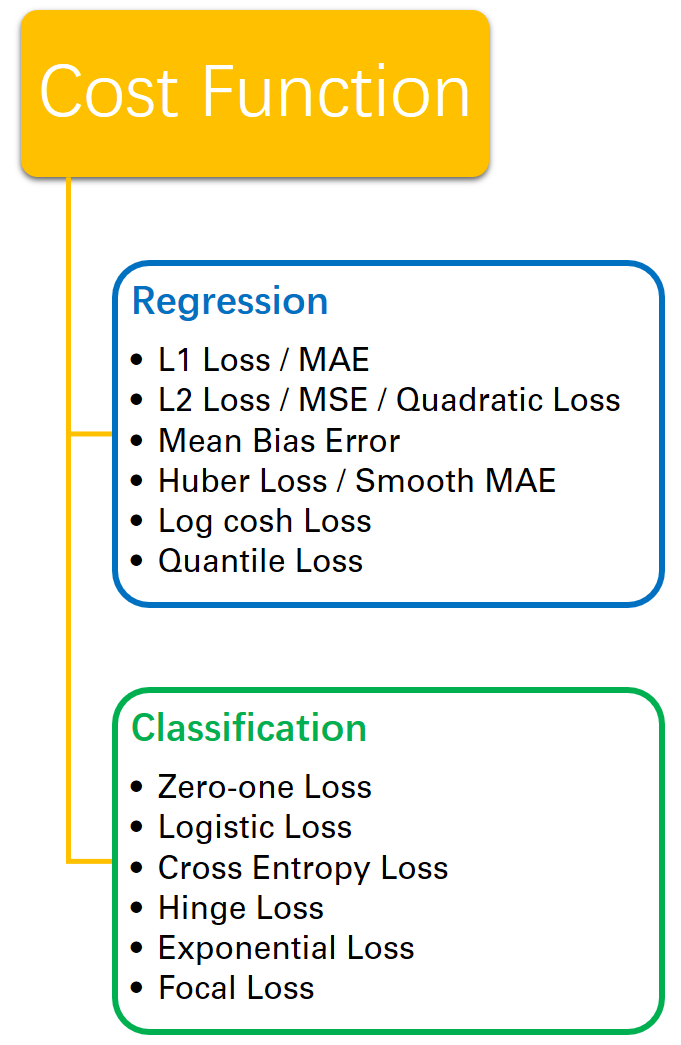y：真实值    y^：预测值    n：样本量

# 2|0回归损失

## 2|1L2损失

MSE=∑ni=1(yi−yi^)2n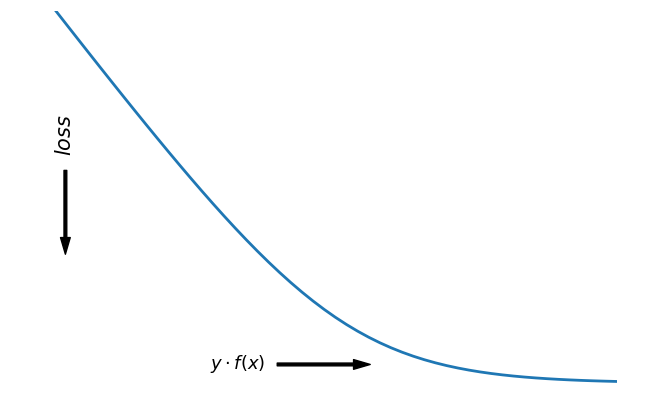sign(f(x))={+1if yf(x)≥0−1if yf(x)<0

## 3|10-1损失 (Zero-one loss)

L(y,f(x))={0ifyf(x)≥01ifyf(x)<0

0-1损失对每个错分类点都施以相同的惩罚，这样那些“错的离谱“ (即margin→−∞)的点并不会收到大的关注，这在直觉上不是很合适。另外0-1损失不连续、非凸，优化困难，因而常使用其他的代理损失函数进行优化。

## 3|2Logistic Loss

L(y,f(x))=log(1+e−yf(x))L(y,f(x))=log⁡(1+e−yf(x))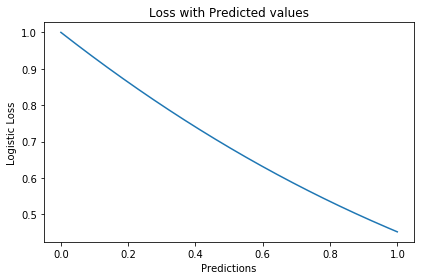logistic Loss为Logistic Regression中使用的损失函数，下面做一下简单证明：

Logistic Regression中使用了Sigmoid函数表示预测概率：

g(f(x))=P(y=1|x)=11+e−f(x)

P(y=−1|x)=1−P(y=1|x)=1−11+e−f(x)=11+ef(x)=g(−f(x))

max(∏i=1mP(yi|xi))=max(∏i=1m11+e−yif(xi))

max(∑i=1mlogP(yi|xi))=−min(∑i=1mlog(11+e−yif(xi)))=min(∑i=1mlog(1+e−yif(xi)))

∏i=1m(P(ti=1|xi))ti((1−P(ti=1|x))1−ti

∑i=1m{−tilogP(ti=1|xi)−(1−ti)log(1−P(ti=1|xi))}

【以上证明是在概率函数确定为Sigmoid的基础上所做的推导，对于更普遍的直接用概率pp表示而进行的推导，详见下一节交叉熵损失。】

## 3|3交叉熵损失（Cross Entropy Loss）

Cross Entropy Loss=−(yilog(yi^)+(1−yi)log(1−yi^))Cross Entropy Loss=−(yilog⁡(yi^)+(1−yi)log⁡(1−yi^))

D(P||Q)=∑x∈XP(x)logP(x)Q(x)=−∑x∈XP(x)logQ(x)−H(P)

【有关熵的相关知识在信息，信息量与信息熵这篇有专门的介绍，这里就不展开详谈了。】

−(yilogP(x;θ)+(1−yi)log(1−P(x;θ)))。

### 负对数似然损失（Negative Log Likelihood）

l(θ)=log∏i=1nPyi(xi;θ)=∑i=1nlogPyi(xi;θ)

logPY(x;θ)=ylogP(x;θ)+(1−y)log(1−P(x;θ))

∑i=1m{−tilogP(ti=1|xi)−(1−ti)log(1−P(ti=1|xi))}

### 交叉熵与Softmax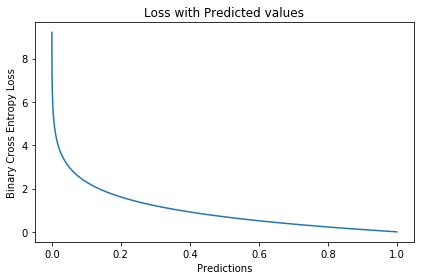Softmax分类器使用的即为交叉熵损失函数，Binary Cross Entropy Loss为二分类交叉熵损失，Categorical Crossentropy为多分类交叉熵损失，当使用多分类交叉熵损失函数时，标签应该为多分类模式，即使用one-hot编码的向量。

P(Y=y|x)={hθ(x)=g(f(x))=11+exp{−f(x)}1−hθ(x)=1−g(f(x))=11+exp{f(x)}y=1y=0

L(y,P(Y=y|x))={log(1+exp{−f(x)})y=1log(1+exp{f(x)})y=0

J(θ)=−1m[∑i=1my(i)loghθ(x(i))+(1−y(i))log(1−hθ(x(i)))]

## 3|4Hinge Loss

L(y)=max(1−yy^)

Hinge loss，中文名铰链损失，也被翻译为“合页损失“”。在机器学习中常用于"最大间隔（maximum-margin）"的分类任务中，如支持向量机SVM。尽管不可微，但它是一个凸函数，因此可以轻而易举地使用机器学习领域中常用的凸优化器。

• 如果y^y<1，则损失为：1−y^y
• 如果y^y≥1，则损失为：0

Hinge loss使得hatyy>1的样本损失皆为0，由此带来了稀疏解，使得SVM仅通过少量的支持向量就能确定最终超平面。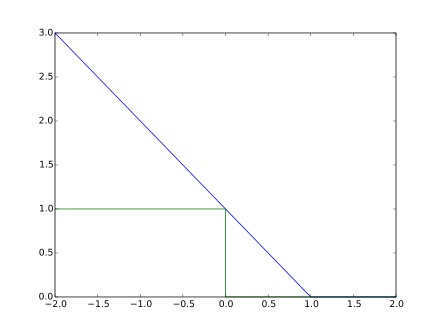### 优化

∂L∂wi={−y⋅xi0ify^y<1otherwise

### 变种

l(y,y′)=max(0,m−y+y′)其中，yy是正样本的得分，y′是负样本的得分，m是margin（自己选一个数）。

l(w,w+,w−)=max(0,1−wT⋅w+ +wT⋅w−)

## 3|5Exponential Loss

L(y,f(x))=e−yf(x)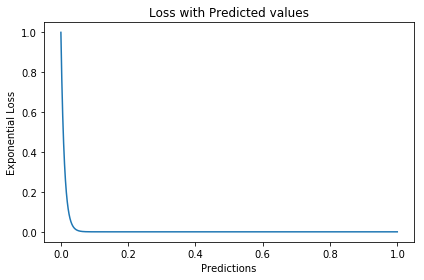argminα,G=∑i=1Nexp[−yi(fm−1(xi)+αG(xi))]

L(y,f(x))=1n∑i=1nexp[−yif(xi)]

minf(x)ExEY|x(e−Yf(x))

f∗(x)=argminf(x)ExEY|x(e−Yf(x))=12logPr(Y=1|x)Pr(Y=−1|x)

f∗(x)估计的是对数几率的一半。当Pr(Y=1|x)>Pr(Y=−1)时， f∗(x)的符号为正，反之为负。于是sign(f∗(x))作为预测值可达贝叶斯最优。这说明了指数函数作为损失函数的合理性。当f(x)为线性函数时，这就是Logistic Regression。

P(x)=Pr(Y=1|x)=11+e−2f(x)

l(Y,P(x))=−Y′logP(x)−(1−Y′)log(1−P(x))=log(1+e−2Yf(x))(1)(2)

f(x)=12logP(y=1|x)P(y=−1|x)

## 3|6Focal Loss

LFL(y)={−α(1−y′)γlogy′−(1−alpha)y′γlog(1−y′)y=1y=0

Focal Loss来自ICCV2017 RBG和Kaiming大神的新作《Focal Loss for Dense Object Detection》。论文详解推荐这篇Focal loss论文详解，介于篇幅，这里只做简单介绍。

Focal loss主要是为了解决one-stage目标检测中正负样本比例严重失衡的问题。该损失函数降低了大量简单负样本在训练中所占的权重，也可理解为一种困难样本挖掘。它是在交叉熵损失函数基础上进行的修改，首先回顾二分类交叉熵损失：

LCE=−ylogy′−(1−y)log(1−y′)={−logy′−log(1−y′)y=1y=0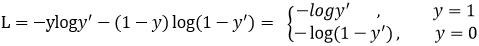y′y′是经过激活函数的输出，所以在0-1之间。可见普通的交叉熵对于正样本而言，输出概率越大损失越小。对于负样本而言，输出概率越小则损失越小。此时的损失函数在大量简单样本的迭代过程中比较缓慢且可能无法优化至最优。那么Focal loss是怎么改进的呢？

LFL={−(1−y′)γlogy′−y′γlog(1−y′)y=1y=0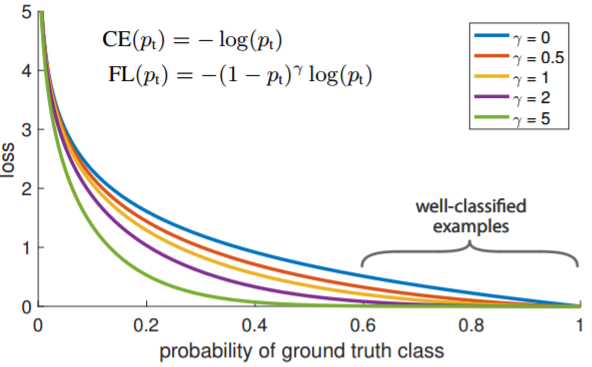LFL={−α(1−y′)γlogy′−(1−α)y′γlog(1−y′)y=1y=0

γ调节简单样本权重降低的速率，当γ为0时即为交叉熵损失函数，当γ增加时，调整因子的影响也在增加。实验发现γ为2是最优。

## 3|7补充解答

### 能不能设计一个分类模型，当使用平方损失时，损失函数关于参数是凸函数？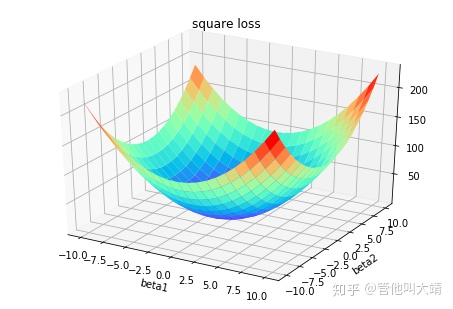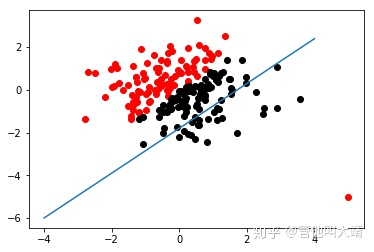f(x;w,b)=⎧⎩⎨1−1wTx+bifwTx+b>1ifwTx+b<−1else

loss(y;f(x))=⎧⎩⎨1−1loss(y;f(x))ifloss(y;f(x))>1ifloss(y;f(x))<−1else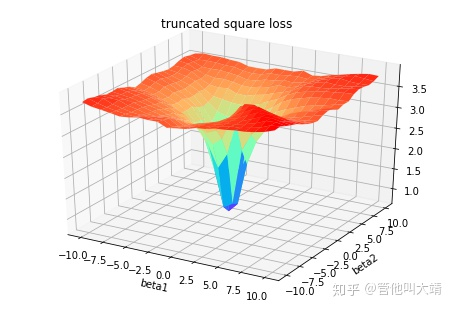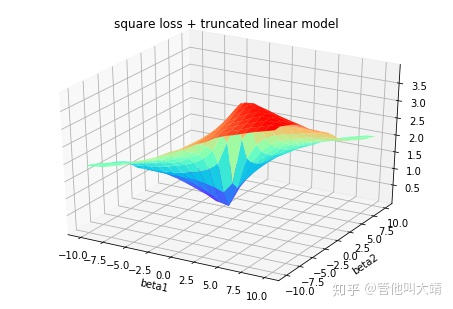（整理自网络）

https://nbviewer.jupyter.org/github/groverpr/Machine-Learning/blob/master/notebooks/05_Loss_Functions.ipynb

https://www.jiqizhixin.com/articles/091202

https://zhuanlan.zhihu.com/p/39239829

https://zhuanlan.zhihu.com/p/35708936

https://zhuanlan.zhihu.com/p/74073096

https://www.zhihu.com/question/319865092

https://blog.csdn.net/zhangjunp3/article/details/80467350

https://www.cnblogs.com/massquantity/p/8964029.html

https://www.pianshen.com/article/3162136463/

https://www.cnblogs.com/king-lps/p/9497836.html03-122477
07-16540

03-19477
05-292562
01-251010
02-28139
08-071695
03-061万+
10-223347
04-032325
11-274144
05-291万+
04-08313
04-032110
08-09798
02-271092
07-28536
12-02564

### “相关推荐”对你有帮助么？

•非常没帮助
•没帮助
•一般
•有帮助
•非常有帮助点击重新获取扫码支付余额充值